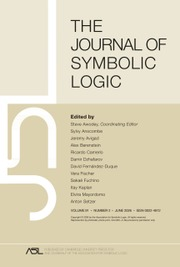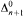HomeThe Journal of Symbolic Logic

# Computable categoricity of trees of finite height

Published online by Cambridge University Press:  12 March 2014

Corresponding

## Abstract

We characterize the structure of computably categorical trees of finite height, and prove that our criterion is both necessary and sufficient. Intuitively, the characterization is easiest to express in terms of isomorphisms of (possibly infinite) trees, but in fact it is equivalent to a-condition. We show that all trees which are not computably categorical have computable dimension ω. Finally, we prove that for every n ≥ 1 in ω, there exists a computable tree of finite height which is Σ30-categorical but not Δn3-categorical

Type
Research Article
Information
The Journal of Symbolic Logic , March 2005 , pp. 151 - 215

## Access options

Get access to the full version of this content by using one of the access options below.

## References

Ash, C. J., Categoricity in hyperarithmetical degrees, Annals of Pure and Applied Logic, vol. 34 (1987), pp. 114.CrossRefGoogle Scholar
Ash, C. J. and Knight, J. F., Computable structures and the hyperarithmetic hierarchy, Elsivier Science, Amsterdam, 2000.Google Scholar
Ash, C. J., Knight, J. F., Mannasse, M., and Slaman, T., Generic copies of countable structures, Annals of Pure and Applied Logic, vol. 42 (1989), pp. 195205.CrossRefGoogle Scholar
Chisholm, J., On intrisically 1-computable trees, unpublished manuscript.Google Scholar
Crossley, J. N., Manaster, A. B., and Moses, M. F., Recursive categoricity and recursive stability, Annals of Pure and Applied Logic, vol. 31 (1986), pp. 191204.CrossRefGoogle Scholar
Downey, R. G., On presentations of algebraic structures, Complexity, logic, and recursion theory (Sorbi, A., editor), Dekker, New York, 1997, pp. 157205.Google Scholar
Downey, R. G. and Jockusch, C. G., Every low Boolean algebra is isomorphic to a recursive one, Proceedings of the American Mathematical Society, vol. 122 (1994), pp. 871880.CrossRefGoogle Scholar
Goncharov, S. S., Autostability and computable families of constructivizations, Algebra and Logic, vol. 14 (1975), pp. 647680 (Russian), 392–409 (English translation).CrossRefGoogle Scholar
Goncharov, S. S., The problem of the number of non-self-equivalent constructivizations, Algebra and Logic, vol. 19 (1980), pp. 401414 (English translation).CrossRefGoogle Scholar
Goncharov, S. S., Groups with a finite number of constructivizations, Soviet Mathematics Doklady, vol. 19 (1981). pp. 5861.Google Scholar
Goncharov, S. S., Nonequivalent constructivizations, Nauka, Novosibirsk, 1982.Google Scholar
Goncharov, S. S., Autostable models and algorithmic dimensions. Handbook of Recursive Mathematics, vol. 1, Elsevier, Amsterdam, 1998.Google Scholar
Goncharov, S. S. and Dzgoev, V. D., Autostability of models, Algebra and Logic, vol. 19 (1980), pp. 4558 (Russian), 28–37 (English translation).Google Scholar
Goncharov, S. S., Lempp, S., and Solomon, R., The computable dimension of ordered abelian groups, Advances in Mathematics, vol. 175 (2003), pp. 102143.CrossRefGoogle Scholar
Goncharov, S. S., Molokov, A. V., and Romanovskii, N. S., Nilpotent groups of finite algorithmic dimension, Siberian Mathematics Journal, vol. 30 (1989), pp. 6368.CrossRefGoogle Scholar
Hirschfeldt, D. R., Khoussainov, B., Shore, R. A., and Slinko, A. M., Degree spectra and computable dimension in algebraic structures, Annals of Pure and Applied Logic, vol. 115 (2002), pp. 71113.CrossRefGoogle Scholar
Khoussainov, B. and Shore, R. A., Computable isomorphisms, degree spectra of relations, and Scott families, Annals of Pure and Applied Logic, vol. 93 (1998), pp. 153193.CrossRefGoogle Scholar
Khoussainov, B., Effective model theory: the number of models and their complexity, Models and computability: Invited papers from Logic Colloquium '97 (Cooper, S. B. and Truss, J. K., editors), London Mathematical Society Lecture Note Series, vol. 259, Cambridge University Press, Cambridge, 1999, pp. 193240.CrossRefGoogle Scholar
Kruskal, J. B., Well quasi-ordering, the tree theorem, and Vázsonyi's conjecture, Transactions of the American Mathematical Society, vol. 95 (1960). pp. 210225.Google Scholar
Kudinov, O. V., An integral domain with finite algorithmic dimension, unpublished manuscript.Google Scholar
Kudinov, O. V., An autostable 1-decidable model without a computable Scott family of ∃ formulas, A Igebra and Logic, vol. 35 (1996), pp. 255260 (English translation).CrossRefGoogle Scholar
LaRoche, P., Recursively presented Boolean algebras, Notices of the American Mathematical Society, vol. 24 (1977), pp. A552, research announcement.Google Scholar
Metakides, G. and Nerode, A., Effective content offield theory, Annals of Mathematical Logic, vol. 17 (1979), pp. 289320.CrossRefGoogle Scholar
Miller, R. G., The Δ20 spectrum of a linear order, this Journal, vol. 66 (2001), pp. 470486.Google Scholar
Miller, R. G., The computable dimension of trees of infinite height, this Journal, vol. 70 (2005). pp. 111141.Google Scholar
Nash-Williams, C. St. J. A., On well-quasi-ordering finite trees, Proceedings of the Cambridge Philosophical Society, vol. 59 (1963), pp. 833835.CrossRefGoogle Scholar
Nurtazin, A. T., Strong and weak constructivizations and enumerable families, Algebra and Logic, vol. 13 (1974), pp. 177184.CrossRefGoogle Scholar
Nurtazin, A. T., Computable classes and algebraic criteria of autostability, thesis, Mathematical Institute of the Siberian Branch of SSSR Academy of Sciences, Novosibirsk, 1974 (Russian).Google Scholar
Remmel, J. B., Recursive isomorphism types of recursive Boolean algebras, this Journal, vol. 46 (1981), pp. 572594.Google Scholar
Remmel, J. B., Recursively categorical linear orderings, Proceedings of the American Mathematical Society, vol. 83 (1981), pp. 387391.CrossRefGoogle Scholar
Simpson, S. G., Nonprovability of certain combinatorial properties of finite trees, Harvey Friedman's research on the foundations of mathematics (Harrington, L. A., Morley, M. D., Scedrov, A., and Simpson, S. G., editors), North-Holland, Amsterdam, 1985, pp. 87117.CrossRefGoogle Scholar
Slaman, T. A., Relative to any nonrecursive set, Proceedings of the American Mathematical Society, vol. 126 (1998), pp. 21172122.CrossRefGoogle Scholar
Soare, R. I., Recursively enumerable sets and degrees, Springer-Verlag, New York, 1987.CrossRefGoogle Scholar
Wehner, S., Enumerations, countable structures, and Turing degrees, Proceedings of the American Mathematical Society, vol. 126 (1998), pp. 21312139.CrossRefGoogle Scholar
White, W., On the complexity of categoricity in computable structures, Mathematical Logic Quarterly, vol. 49 (2003), no. 6, pp. 603614.CrossRefGoogle Scholar

### Full text views

Full text views reflects PDF downloads, PDFs sent to Google Drive, Dropbox and Kindle and HTML full text views.

Total number of HTML views: 0
Total number of PDF views: 11 *
View data table for this chart

* Views captured on Cambridge Core between September 2016 - 17th January 2021. This data will be updated every 24 hours.

Hostname: page-component-77fc7d77f9-kstv4 Total loading time: 0.26 Render date: 2021-01-17T14:18:37.713Z Query parameters: { "hasAccess": "0", "openAccess": "0", "isLogged": "0", "lang": "en" } Feature Flags last update: Sun Jan 17 2021 14:03:35 GMT+0000 (Coordinated Universal Time) Feature Flags: { "metrics": true, "metricsAbstractViews": false, "peerReview": true, "crossMark": true, "comments": true, "relatedCommentaries": true, "subject": true, "clr": true, "languageSwitch": true, "figures": false, "newCiteModal": false, "shouldUseShareProductTool": true, "shouldUseHypothesis": true, "isUnsiloEnabled": true }

# Send article to Kindle

Note you can select to send to either the @free.kindle.com or @kindle.com variations. ‘@free.kindle.com’ emails are free but can only be sent to your device when it is connected to wi-fi. ‘@kindle.com’ emails can be delivered even when you are not connected to wi-fi, but note that service fees apply.

Find out more about the Kindle Personal Document Service.

Computable categoricity of trees of finite height
Available formats
×

# Send article to Dropbox

To send this article to your Dropbox account, please select one or more formats and confirm that you agree to abide by our usage policies. If this is the first time you use this feature, you will be asked to authorise Cambridge Core to connect with your <service> account. Find out more about sending content to Dropbox.

Computable categoricity of trees of finite height
Available formats
×

# Send article to Google Drive

To send this article to your Google Drive account, please select one or more formats and confirm that you agree to abide by our usage policies. If this is the first time you use this feature, you will be asked to authorise Cambridge Core to connect with your <service> account. Find out more about sending content to Google Drive.

Computable categoricity of trees of finite height
Available formats
×
×